The International Information Center for Structural Engineers

Tuesday, 12 January 2016 12:16cat

## Calculation Example: Calculate the Change in Length of a Rod loaded in extension

Written by  TheStructuralEngineer.info

The rod of the picture is loaded with the force P2 which is uniformly distributed on Section D where applied.

If the material of the rod is homogeneous and elastic with Elastic Modulus E find the variation in length of the rod. The area of the cross section in part AC is F1 while CD is F2.Solution

We calculate the reaction RA at the rigid end connection A from the equilibrium of forces.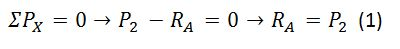The rod doesn’t have constant area of cross sectional area so we examine parts AC and CD.

For AC the cross sectional area F1 is loaded with axial force equal to RA. So the uniform stress ?1 and deformation ?1 are:Since the material is homogeneous and elastic Hooke's Law is applied for section AC.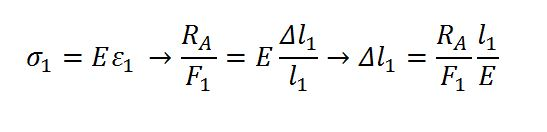(2)

For section CD the axial force P2 is: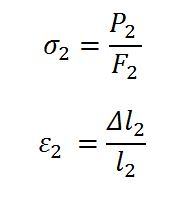And Hooke's Law: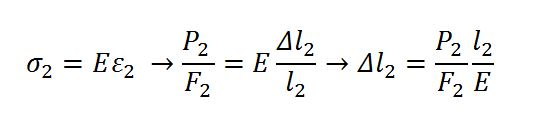(3)

From equations (1),(2),(3) we have the total variation of length of the rod is: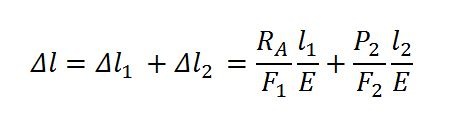Where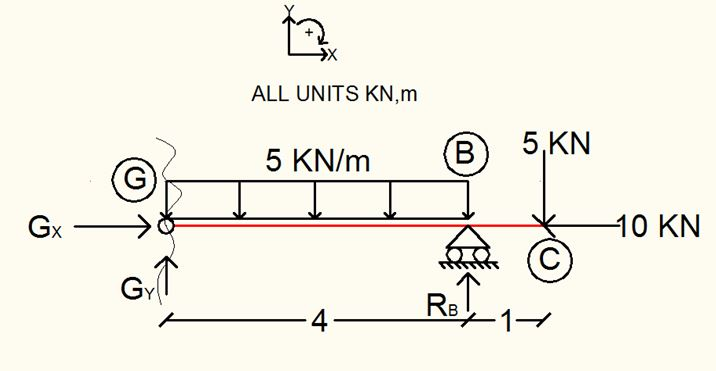• Default
• Title
• Date
• Random
• ## Petrochemical Sump and Pipe Support by Hayward Baker

Hayward Baker worked on a sheet pile & helical pile installation in restricted access conditions in a busy petrochemical facility.